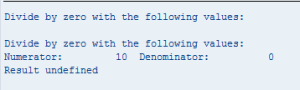# Resumable exceptions

Resume code execution after an exception has been thrown can be very useful in several different scenarios (i.e. processing multiple materials and you want to process all but skip only those where there’s an error)

This can be solved quite easily using resumable exceptions

First we define our exception class (can be global – defined in DDIC or locally defined). Nothing special needs to be specified here.

```CLASS lcx_my_exception DEFINITION
INHERITING FROM cx_static_check.
PUBLIC SECTION.
DATA:
message TYPE char50.

METHODS:
constructor
IMPORTING
message TYPE char50 OPTIONAL.
ENDCLASS.                    "LCX_MY_EXCEPTION DEFINITION

CLASS lcx_my_exception IMPLEMENTATION.
METHOD constructor.
super->constructor( ).
me->message = message.
ENDMETHOD.                    "CONSTRUCTOR
ENDCLASS.                    "LCX_MY_EXCEPTION IMPLEMENTATION```

I will demonstrate both the classic exception and resumable exception on a division operation with methods DIVIDE and DIVIDE_RESUMABLE

```CLASS lcl_math IMPLEMENTATION.
METHOD divide_resumable.
TRY.
IF i_denominator = 0.
RAISE RESUMABLE EXCEPTION TYPE lcx_my_exception
EXPORTING
message = 'Divide by zero with the following values:'.
ELSE.
result = i_numerator / i_denominator.
ENDIF.

WRITE:/ 'Numerator:', i_numerator,
'Denominator:', i_denominator.
ENDTRY.
ENDMETHOD.                    "divide_resumable

METHOD divide.
IF i_denominator = 0.
RAISE EXCEPTION TYPE lcx_my_exception
EXPORTING
message = 'Divide by zero with the following values:'.
ELSE.
result = i_numerator / i_denominator.
ENDIF.

* !!! NOT RESUMABLE !!!
* The following line will never be hit when denominator = 0
WRITE:/ 'Numerator:', i_numerator,
'Denominator:', i_denominator.
ENDMETHOD.                    "divide
ENDCLASS.                    "lcl_math IMPLEMENTATION```

Note the difference in raising commands:

Method DIVIDE_RESUMABLE

`RAISE RESUMABLE EXCEPTION TYPE lcx_my_exception `

Method DIVIDE

`RAISE EXCEPTION TYPE lcx_my_exception`

Here follows a demo program that calls the division operation in both resumable and non-resumable form:

```DATA:
gr_ex    TYPE REF TO lcx_my_exception,
g_result TYPE anzh2,
g_err    TYPE abap_bool.

START-OF-SELECTION.
TRY.
g_result = lcl_math=>divide(
i_numerator = 10
i_denominator = 0
).

*     !!! NOT RESUMABLE !!!
*     The following CASE block will never be executed when denominator = 0
CASE g_err.
WHEN space.
WRITE:/ 'Result:', g_result.
WHEN OTHERS.
WRITE:/ 'Result undefined'.
ENDCASE.

CATCH lcx_my_exception INTO gr_ex.
g_err = abap_true.
WRITE:/ gr_ex->message.
ENDTRY.

SKIP 1.
CLEAR:
g_err,
gr_ex.

TRY.
g_result = lcl_math=>divide_resumable(
i_numerator = 10
i_denominator = 0
).

CASE g_err.
WHEN space.
WRITE:/ 'Result:', g_result.
WHEN OTHERS.
WRITE:/ 'Result undefined'.
ENDCASE.

CATCH BEFORE UNWIND lcx_my_exception INTO gr_ex.
WRITE:/ gr_ex->message.

IF gr_ex->is_resumable = abap_true.
g_err = abap_true.
RESUME.
ENDIF.
ENDTRY.```

Note the difference in catch commands:

Method DIVIDE_RESUMABLE (calls RESUME which returns to previous processing right after command which raised this exception)

```CATCH BEFORE UNWIND lcx_my_exception INTO gr_ex.
...
RESUME```

Method DIVIDE

`CATCH lcx_my_exception INTO gr_ex.`

And the output of this simple from is shown on follwing screenshot/You must be Logged on to comment or reply to a post.
•thats a good example thanks mate!!

great help

•Hello Miroslav,

I got the idea of Resumable exception but not clear with its practical utility.

If we take material creation example  then say we have a code like this

loop at lt_mat in ls_mats.

TRY.

Call METHOD.

CATCH.

ENDTRY.

endloop.

Even if the exception occurs in any of iteration them then processing will be continue for rest of materials.

Have you ever seen a real time scenario with Resumable exception.

•Former Member Post author

Hello Jitendra,

You are correct in this special case.

But imagine you have a main process thread, which calls a function module FM1 and this calls another function module FM2.

In FM2 there is a RESUMABLE execption raised and it’s not handled within FM2 – it’s propagated to FM1.

Iin FM1 it is not handled too so it’s propagated to main process thread.

In this main thread you handle/catch the resumable exception and you can decide on this TOP level whether you want to continue processing the rest the FM2 code or not.

So if I align it to your example – let’s assume your CALL METHOD raises an exception.

But you’d like to handle the exception somehow and then continue processing with remainig code in the CALL METHOD from place where the resumable exception was thrown.

Using your TRY-CATCH-ENTRY approach you are NOT able to achieve this.

Using Resumable exception, you are able to do it.

Br

— Miroslav